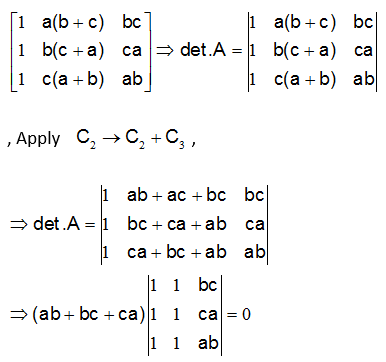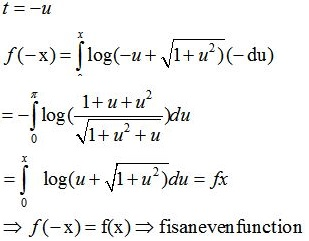# CBSE 12TH MATHEMATICS - Online Test

Q1. Differential equations are equations containing functions y = f(x), g(x) and
Explaination / Solution:

Differential equations are equations containing functions y = f(x), g(x) and derivatives of y with respect to x.

Q2. Direction cosines of a line are
Explaination / Solution:

a line when intersect another line or axis two kind of angle form ,as an assumption we take positive side of axis, to define the direction of line we take angle made from all three axis.and we take cos not other trigonometric function like sin,tan because we can define Direction cosines of a line are coefficient of i,j,k of a unit vector along that line.

Q3. The area of ellipse  is
Explaination / Solution:

Area of standard ellipse is given by :πab.

Q4. If A and B are symmetric matrices of the same order, then
Explaination / Solution:

If A and B are symmetric matrices of the same order, then AB + BA is always a symmetric matrix.

Q5. The instantaneous rate of change at t = 1 for the function f (t) =  is
Explaination / Solution:

f(1)=e1+e1=0
Q6. Let A =, then Det. A is
Explaination / Solution:( since C1 and C2 are identical)

Q7. A linear programming problem is one that is concerned with
Explaination / Solution:

A linear programming problem is one that is concerned with finding the optimal value (maximum or minimum) of a linear function of several variables .

Q8. The function  is
Explaination / Solution:Q9. Vector has
Explaination / Solution:

A vector has both magnitude as well as direction.

Q10. A coin is tossed three times, if E : head on third toss , F : heads on first two tosses. Find P(E|F)
Explaination / Solution: